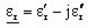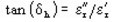# Isotropic / anisotropic material: linear approximation (with losses)

## Presentation

This model defines a D(E) law of linear behavior for an isotropic or anisotropic material.

It takes into account the dielectric losses in harmonic regimes (Steady state AC Electric Application)

## Mathematical model

This model is a straight line.

The corresponding mathematical formula is written:

D(E)= ε0εrE

where:

• ε0 is the permittivity of vacuum; ε0 = 1/(36 π). 10-9 [F/m]
• εr is the relative permittivity of the material in its complex form:
•and## Anisotropic material

For an anisotropic material, this linear model consists of a group of three straight lines.